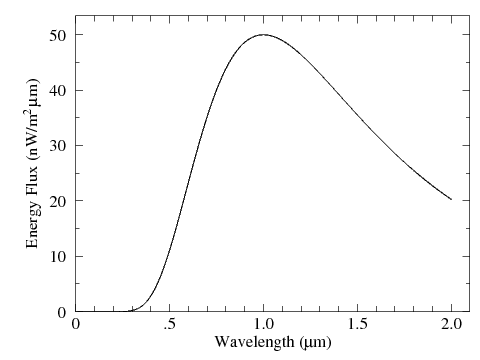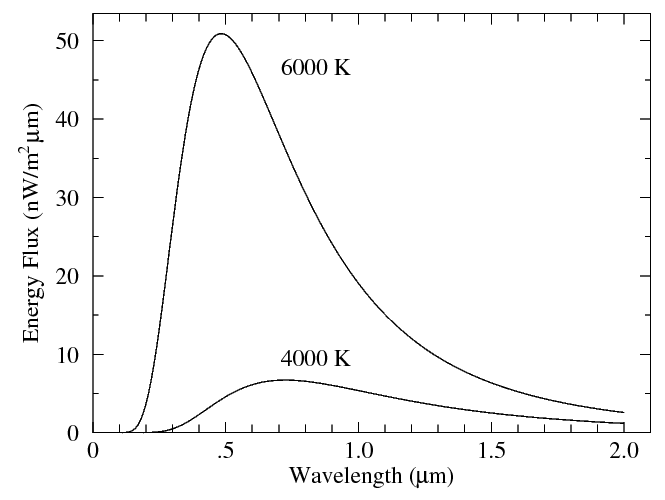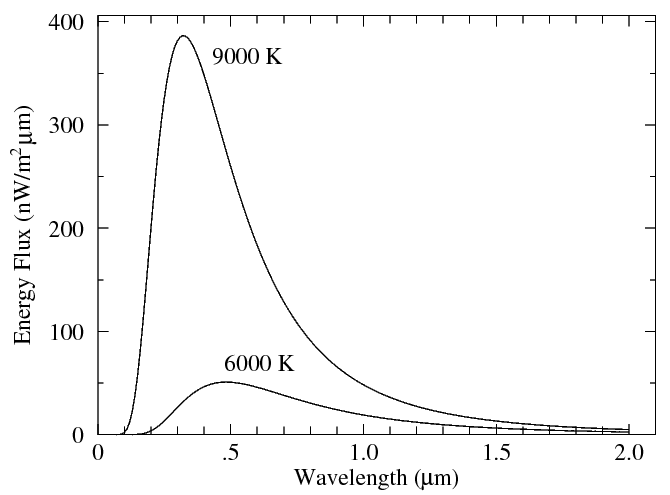## Blackbody Spectra QuizLast four digits of your student ID number:

Note: If the above fields are not filled in, grading will not occur!

Select the letter of the single best answer. Each answer is worth 1 point.

If you are unsure how to use this form, try this example.

The above is an attempt to render the visible spectrum on your monitor. Different wavelengths of light (λ, from .380 μm to .750 μm) are seen by the human eye as different colors (V=violet, B=blue, G=green, Y=yellow, O=orange, R=red). For the following questions, you should assume blue light corresponds to a wavelength of .450 μm, green light corresponds to a wavelength of .550 μm, and red light corresponds to a wavelength of .700 μm. Of course there is a continuum of color variation across the color spectrum.

The following plots (called spectra, or spectral energy distributions) display the amount of light energy as a function of wavelength. The y-axis has been labeled "Energy Flux". Properly speaking it is "spectral irradiance" but is often labeled "Intensity" in textbooks. You can think of it as the amount of light energy (watts) of a particular wavelength (μm) hitting a square meter (m2). When we need a symbol to denote this quantity we will denote it as Fλ.

This quiz deals exclusively with ideal "blackbody" spectra. The light you would receive from such an object just depends on the object's radius, distance from you, and temperature. The next quiz will deal with more complex spectra, like that from the Sun.1. The amount of blue light in this spectra is most closely:

1. .45 μm
2. 50 nW/m2 μm
3. 1.0 μm
4. 6 nW/m2 μm
5. 20 nW/m2 μm
```
A B C D E

```

2. The wavelength that produces the maximum amount of light (λmax) in this spectra is most closely:

1. .45 μm
2. 50 nW/m2 μm
3. .7 μm
4. 1 μm
5. 2 μm
```
A B C D E

```

3. Let Fλ(B) denote the amount of blue light and Fλ(R) denote the amount of red light. Which of the following is correct?

1. Fλ(B) < Fλ(R)
2. Fλ(B) = Fλ(R)
3. Fλ(B) > Fλ(R)
```
A B C

```

4. The ratio of the amount of blue light to the amount of red light, Fλ(B)/Fλ(R), is most closely:

1. .2
2. .6
3. 1.5
4. 6
5. 10
```
A B C D E

```

5. Most of this star's light is:

1. infrared light
2. visible light
3. ultraviolet light
4. X-ray light
```
A B C D

```6. The spectra labeled I is identical to the first spectra; the spectra labeled II is from an star that is closer to Earth than the star in I, but is otherwise identical. In comparing the two spectra, which of the following quantities would be different for the two stars?

1. λmax
2. the ratio: Fλ(B)/Fλ(R)
3. Fλ(B)
4. more than one of the above
5. none of the above
```
A B C D E

```

These two spectra are from two stars that are identical (same radius, same distance from Earth, etc.) except that one has a surface temperature similar to the Sun's (6000 K) while the other is cooler: 4000 K.7. Which star produces the most visible light?

1. 4000 K
2. 6000 K
3. they produce the same amount of visible light
```
A B C

```

8. Which star produces the most infrared light?

1. 4000 K
2. 6000 K
3. they produce the same amount of infrared light
```
A B C

```

9. For the 6000 K star:

1. Fλ(B) < Fλ(R)
2. Fλ(B) = Fλ(R)
3. Fλ(B) > Fλ(R)
```
A B C

```

10. For the 4000 K star:

1. Fλ(B) < Fλ(R)
2. Fλ(B) = Fλ(R)
3. Fλ(B) > Fλ(R)
```
A B C

```

11. Which if the following statements is true?

1. Since for the 4000 K star λmax≅0.7 μm, the 4000 K star produces more red light than does the 6000 K star.
2. The higher temperature star has the larger value of λmax.
3. If the 6000 K star had a smaller radius or was at a greater distance from the Earth, the ratio Fλ(B)/Fλ(R) would be smaller.
4. More than one of the above
5. None of the above.
```
A B C D E

```

These two spectra are from two stars that are identical (same radius, same distance from Earth, etc.) except that one has a surface temperature similar to the Sun's (6000 K) while the other is hotter: 9000 K.12. Which star produces the most visible light?

1. 9000 K
2. 6000 K
3. they produce the same amount of visible light
```
A B C

```

13. Which star produces the most infrared light?

1. 9000 K
2. 6000 K
3. they produce the same amount of infrared light
```
A B C

```

14. For the 9000 K star:

1. Fλ(B) < Fλ(R)
2. Fλ(B) = Fλ(R)
3. Fλ(B) > Fλ(R)
```
A B C

```

15. Which if the following statements is true?

1. The 6000 K star could outshine the 9000 K star, but it would have to be closer to the Earth and/or have a larger radius then the 9000 K star.
2. If the 6000 K star had a larger radius or was closer to the Earth, the ratio Fλ(B)/Fλ(R) would be unchanged.
3. In both this spectra and the previous, the higher temperature star had the smaller value of λmax.
4. All of the above
5. Two the above.
6. None of the above
```
A B C D E  F

```16. The spectra labeled I is identical to the first spectra; the spectra labeled II is from an unrelated star that happens have about the same bolometric (i.e., summed over all wavelengths) light flux as star I. In comparing the two spectra, which of the following quantities would be different?

1. λmax
2. the ratio: Fλ(B)/Fλ(R)
3. both of the above
4. none of the above
```
A B C D

```

17. Which star produces the most infrared light?

1. I
2. II
3. they produce the same amount of infrared light
```
A B C

```

18. Which star produces the most visible light?

1. I
2. II
3. they produce the same amount of visible light
```
A B C

```

19. For star II: the ratio of the amount of blue light to the amount of red light, Fλ(B)/Fλ(R), is most closely:

1. .2
2. .6
3. 1.2
4. 6
5. 100
```
A B C D E

```

The following questions ask how the below spectra would change if the star changed20. It the star were the same except for an enlarged radius, the spectra would become:1. None of the above
```
A B C D E

```

21. If the star were the same except located further from the Earth, the spectra would become:1. None of the above
```
A B C D E

```

22. If the star were the same except for a reduced temperature, the spectra would become:1. None of the above
```
A B C D E

```

23. If the star were the same except for an increased temperature, the spectra would become:1. None of the above
```
A B C D E

```

24. If the star were the same except: an increased temperature and at a greater distance from the Earth, the spectra would become:1. None of the above
```
A B C D E

```